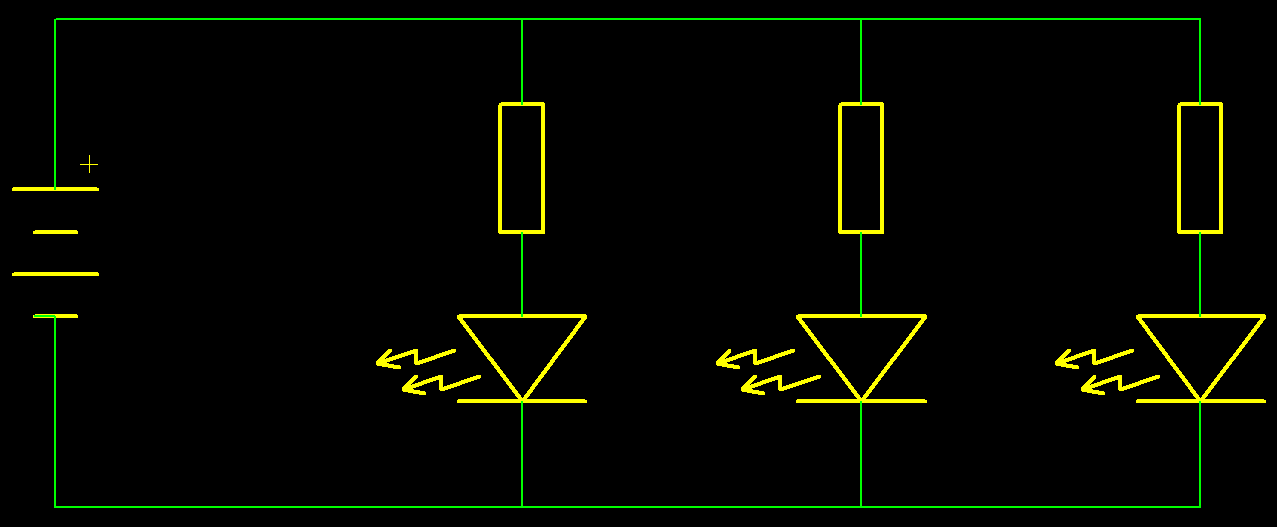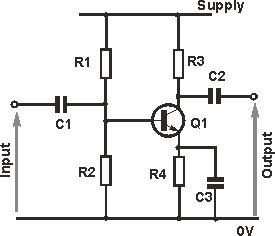# PTCL Electronics

With circuits of resistors, capacitors and inductors as the primary components, there are some simple circuits that can be made.

The first is called series circuits. This is where the lead of one connected to the lead of only one other lead. This typically would have a power supply or signal generator connected into the loop.

The second type called parallel circuits. This is were all of one end of the components are connected together.

Both types of those simple circuits can have parts of the other style within the circuit, such as having a pair of resistors in parallel in a series circuit to reduce the value of the resistor, or a pair of resistors in series in a parallel circuit to increase the resistance of the particular loop.

Where the complication arises with whether the circuit is a series or parallel circuit is when it contains other components which have aspects of both circuits. The power supply is connected in parallel, whilst the signal is connected in series. In this situation we generally stop calling it a series or parallel circuit and start calling it an oscillator circuit or amplifier circuit.

The circuit below contains a simple circuit containing both a parallel aspect and series aspect.On the left of the circuit is a battery. This supplies the power to the rest of the circuit, and is in series with the rest of the components.

There are then 3 identical parallel aspects. Each of those branches has a resistor and LED in series. The resistor is there to allow greater control of the brightness.

The circuit (below) is a basic amplification circuit. The input and output have a capacitor in series with them. The resistor R4 is in parallel with the capacitor C3, and in series with the transistor (Q1). This style is typically not called a series or parallel circuit.